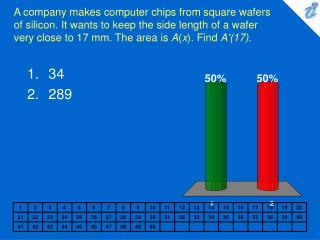DownloadDownload Presentation34 289

# 34 289

Télécharger la présentation## 34 289

- - - - - - - - - - - - - - - - - - - - - - - - - - - E N D - - - - - - - - - - - - - - - - - - - - - - - - - - -
##### Presentation Transcript

1. A company makes computer chips from square wafers of silicon. It wants to keep the side length of a wafer very close to 17 mm. The area is A(x). Find A'(17). • 34 • 289

2. The mass of the part of a metal rod that lies between its left end and a point x meters to the right is {image} .&#160; Find the linear density when x is 4 m. • 16 • 32 • 64

3. 1. 2. 3. Newton's Law of Gravitation says that the magnitude F of the force exerted by a body of mass m on a body of mass M is {image} .&#160;&#160;Find {image} . • {image} • {image} • {image}

4. Suppose that the cost, in dollars, for a company to produce x pairs of jeans is {image} . Find &#160; {image} . • \$58.44 • \$27.08 • \$298.44 • \$54.24

5. The gas law for an ideal gas at absolute temperature T (in kelvins), pressure P (in atmospheres), and volume V (in liters) is PV = nRT, where n is the number of moles of the gas and R = 0.0821 is the gas constant. Suppose that, at a certain instant, P = 7 atm and is increasing at a rate of 0.10 atm/min and V = 10L and is decreasing at a rate of 0.15 L/min. Find the rate of change of T with respect to time at that instant if n = 10 moles. • -0.061 • -0.041 • 2.497